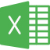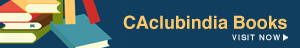### Inserting a Blank Columninserting a blank column after every column...

### Max and Minimum use with conditionMax and minimum with one condititon...

### Inserting a Blank RowHow to insert a blank row ...Advance filter trick by changing formating...

### Extract Value below the Cell Vlookup Findsextract Value below the Cell Vlookup Finds...

### Arranging of Data in ExcelArranging of Data With the help of word...

### Use of Nested if condition for CA Final Examination ResultHere i used nested if use condition with "And" & "Or" Word....

### Use of sumifs with if conditionUse of Sumifs with If condition and one column merged....

### Pivot Table Settingwe can use pivot table value in formula as normal cell....

### Vlookup used in Two dimensionsUse of Vlook up in two dimensions using Match....

### Use of VLookup Function in More than one sheetUSE OF VLOOK UP FUNCTION IN MORE THAN ONE SHEET...

### Use of Indirect Function for ConsolidationConsolidation of Data using Vlookup or Index and IF Error, Match & Indirect....

### Round function of excel with possible aspectComplete understanding of Round function of Excel....More Options »

﻿